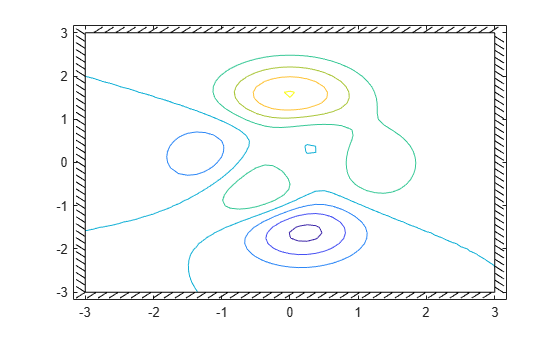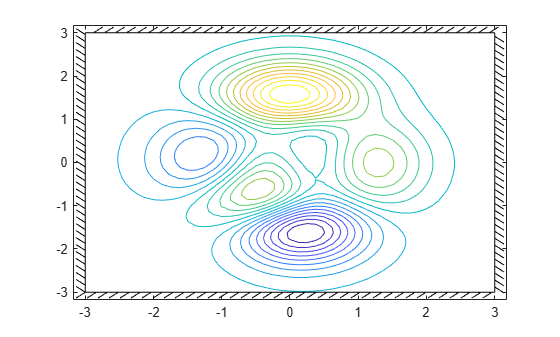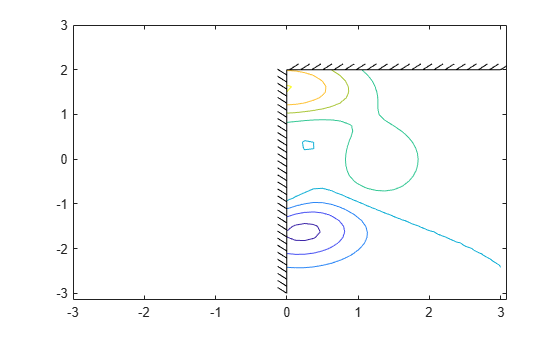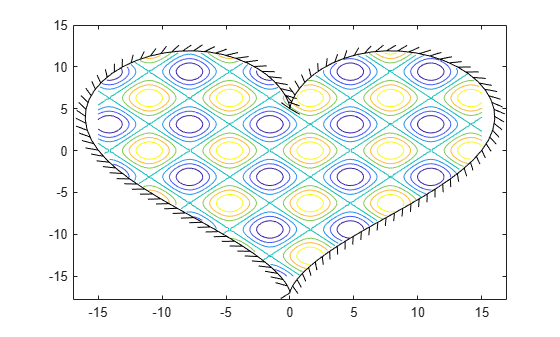# altitudeEnvelopeContour

Draw altitude envelope contour plot

Since R2021b

## Syntax

``altitudeEnvelopeContour(loadfactor)``
``altitudeEnvelopeContour(airspeed,altitude,loadfactor)``
``altitudeEnvelopeContour(___,levels)``
``altitudeEnvelopeContour(___,LineSpec)``
``altitudeEnvelopeContour(___,Name,Value)``
``altitudeEnvelopeContour(ax,___)``
``[c,h,bline] = altitudeEnvelopeContour(___)``

## Description

### Draw Contour Plots with Load Factors and Meshes

example

````altitudeEnvelopeContour(loadfactor)` draws a contour plot of the `loadfactor` matrix in the x-y plane. This function is based on the MATLAB® `contour` function. The x-coordinates of the vertices correspond to the column indices of `loadfactor` and the y-coordinates correspond to the row indices of `loadfactor`. The contour automatically chooses the contour levels. `altitudeEnvelopeContour(airspeed,altitude,loadfactor)` draws a contour plot of the `loadfactor` matrix using vertices from the mesh that `airspeed` and `altitude` define. ```

### Draw Contour Plots with Customizations

example

````altitudeEnvelopeContour(___,levels)` plots an altitude envelope contour specified by the desired levels `levels`.`altitudeEnvelopeContour(___,LineSpec)` plots an altitude envelope contour specified by the desired line spec `LineSpec`.`altitudeEnvelopeContour(___,Name,Value)` plots an altitude envelope contour specified by one or more `Name,Value` arguments.`altitudeEnvelopeContour(ax,___)` draws an altitude contour plot onto the axes ax.```

### Return Matrix, Contour Object, and Boundary Line Object

example

````[c,h,bline] = altitudeEnvelopeContour(___)` returns contour matrix `c`, a contour object `h`, and a vector of `boundaryline` objects `b`. To label the plot, use the `c` and `bs` arguments as inputs to the `clabel` function when using the `LabelSpacing` property. TipFor more information on the contour matrix, see the Contour Properties property for contour objects. ```

## Examples

collapse all

Plot an altitude envelope contour with default levels and boundaries.

```[speed,alt,loadfactor] = peaks(); altitudeEnvelopeContour(speed,alt,loadfactor)```Plot an altitude envelope contour with 20 levels and default boundaries.

```[speed,alt,loadfactor] = peaks(); altitudeEnvelopeContour(speed,alt,loadfactor,20)```Plot an altitude envelope contour with constant boundary lines, and turn off boundary line intersection clipping.

```[speed,alt,loadfactor] = peaks(); altitudeEnvelopeContour(speed,alt,loadfactor,... "MinimumSpeed",0,"MaximumAltitude",2,"ResolveBoundary","off")```Plot an altitude envelope contour with custom boundary data. Return line objects and boundary line objects in `c`, `h`, and `b`.

```x = linspace(-15,15); y = linspace(-15,15); [X,Y] = meshgrid(x,y); Z = sin(X)+cos(Y); t = linspace(2*pi,0); boundaryX = 16*sin(t).^3; boundaryY = 13*cos(t) - 5*cos(2*t) - 2*cos(3*t) - cos(4*t); [c,h,b]=altitudeEnvelopeContour(X,Y,Z,... "BoundaryXData",boundaryX,"BoundaryYData",boundaryY);```## Input Arguments

collapse all

Load Factor for each airspeed and altitude, specified as a numeric matrix typically in g's.

Data Types: `double`

Aircraft airspeed for each corresponding index in `loadfactor`, specified as a numeric vector or matrix.

Data Types: `double`

Aircraft altitude for each corresponding index in `loadfactor`, specified as a numeric vector or matrix.

Data Types: `double`

Levels for which to develop contour lines, specified as a scalar or vector.

• If `levels` is a scalar, `levels` specifies the number of contour lines, and the contour levels are chosen automatically by `contour`.

• If `levels` is a vector, `levels` specifies the number and levels of contour lines to plot.

Tip

To draw the contours at one height (k), specify levels as a two-element row vector ```[k k]```.

Example: `[2 3]`

Data Types: `double`

Line style, marker, and color, specified as a string scalar or character vector containing symbols. The symbols can appear in any order. You do not need to specify all three characteristics (line style, marker, and color). For example, if you omit the line style and specify the marker, then the plot shows only the marker and no line.

Example: `"--or"` is a red dashed line with circle markers.

Line StyleDescriptionResulting Line
`"-"`Solid line`"--"`Dashed line`":"`Dotted line`"-."`Dash-dotted lineMarkerDescriptionResulting Marker
`"o"`Circle`"+"`Plus sign`"*"`Asterisk`"."`Point`"x"`Cross`"_"`Horizontal line`"|"`Vertical line`"square"`Square`"diamond"`Diamond`"^"`Upward-pointing triangle`"v"`Downward-pointing triangle`">"`Right-pointing triangle`"<"`Left-pointing triangle`"pentagram"`Pentagram`"hexagram"`HexagramColor NameShort NameRGB TripletAppearance
`"red"``"r"``[1 0 0]``"green"``"g"``[0 1 0]``"blue"``"b"``[0 0 1]``"cyan"` `"c"``[0 1 1]``"magenta"``"m"``[1 0 1]``"yellow"``"y"``[1 1 0]``"black"``"k"``[0 0 0]``"white"``"w"``[1 1 1]`Valid axes, specified as a scalar handle. By default, this function plots to the current axes, obtainable with the `gca` function.

Data Types: `double`

### Name-Value Arguments

Specify optional pairs of arguments as `Name1=Value1,...,NameN=ValueN`, where `Name` is the argument name and `Value` is the corresponding value. Name-value arguments must appear after other arguments, but the order of the pairs does not matter.

Before R2021a, use commas to separate each name and value, and enclose `Name` in quotes.

Example: `"MinimumSpeed",0`

Note

The properties listed here are only a subset. For a full list, see Contour Properties.

Minimum altitude boundary, specified as a numeric scalar or n-by-2 matrix.

• If `MinimumAltitude` is a scalar, `MinimumAltitude` specifies a horizontal line that intersects with the X limits of the axes or with the intersection of `MinimumSpeed` and `MaximumSpeed`.

• If `MinimumAltitude` is an n-by-2 matrix, each row is an `airspeed` and `altitude` point of the boundary.

The function marks `loadfactor` values below `MinimumAltitude` as NaN.

Data Types: `double`

Maximum altitude boundary, specified as a numeric scalar or n-by-2 matrix.

• If `MaximumAltitude` is a scalar, `MaximumAltitude` specifies a horizontal line that intersects with the X limits of the axes or with the intersection of `MinimumSpeed` and `MaximumSpeed`.

• If `MaximumAltitude` is an n-by-2 matrix, each row is an `airspeed` and `altitude` point of the boundary.

The function marks `loadfactor` values above `MaximumAltitude` as NaN.

Data Types: `double`

Minimum speed boundary, specified as a numeric scalar or n-by-2 matrix.

• If `MinimumSpeed` is a scalar, `MinimumSpeed` specifies a vertical line that intersects with the Y limits of the axes or with the intersection of `MinimumAltitude` and `MaximumAltitude`.

• If `MinimumSpeed` is an n-by-2 matrix, each row is an `airspeed` and `altitude` point of the boundary.

The function marks `loadfactor` values behind `MinimumSpeed` as NaN.

Data Types: `double`

Maximum speed boundary, specified as a numeric scalar or n-by-2 matrix.

• If `MaximumSpeed` is a scalar, `MaximumSpeed` specifies a vertical line that intersects with the Y limits of the axes or with the intersection of `MinimumAltitude` and `MaximumAltitude`.

• If `MaximumSpeed` is an n-by-2 matrix, each row is an `airspeed` and `altitude` point of the boundary.

The function marks as NaN `loadfactor` values in front of `MaximumSpeed`.

Data Types: `double`

Boundary line X data, specified as a numeric vector.

Data Types: `double`

Boundary line Y data, specified as a numeric vector.

Data Types: `double`

Contour data display, specified as:

• `'on'` — Remove the contour data outside the specified boundary lines.

• `'off'` — Show all contour data and specified boundary lines.

Data Types: `double` | `logical` | `string`

Boundary line intersection and enclosure, specified as:

• `'on'` — Resolve boundary line segments to form a closed boundary around the line intersection points. Boundary data points outside the resolved boundary are removed. This method produces a well-formed boundary, but does not allow infinite limits or unconnected boundary lines.

• `'off'` — Do not resolve boundary line segments. This method leaves the boundary line data unmodified to allow infinite limits and unconnected boundary lines.

Tip

This method might produce a malformed boundary that affects the `ClipContour` behavior.

Data Types: `double` | `logical` | `string`

## Output Arguments

collapse all

Contour, returned as a numeric matrix.

Contour graphics object, returned as a scalar.

One or more boundary line objects, returned as a scalar or a vector. These are unique identifiers, which you can use to query and modify properties of a specific chart line. For a list of properties, see Line Properties.

collapse all